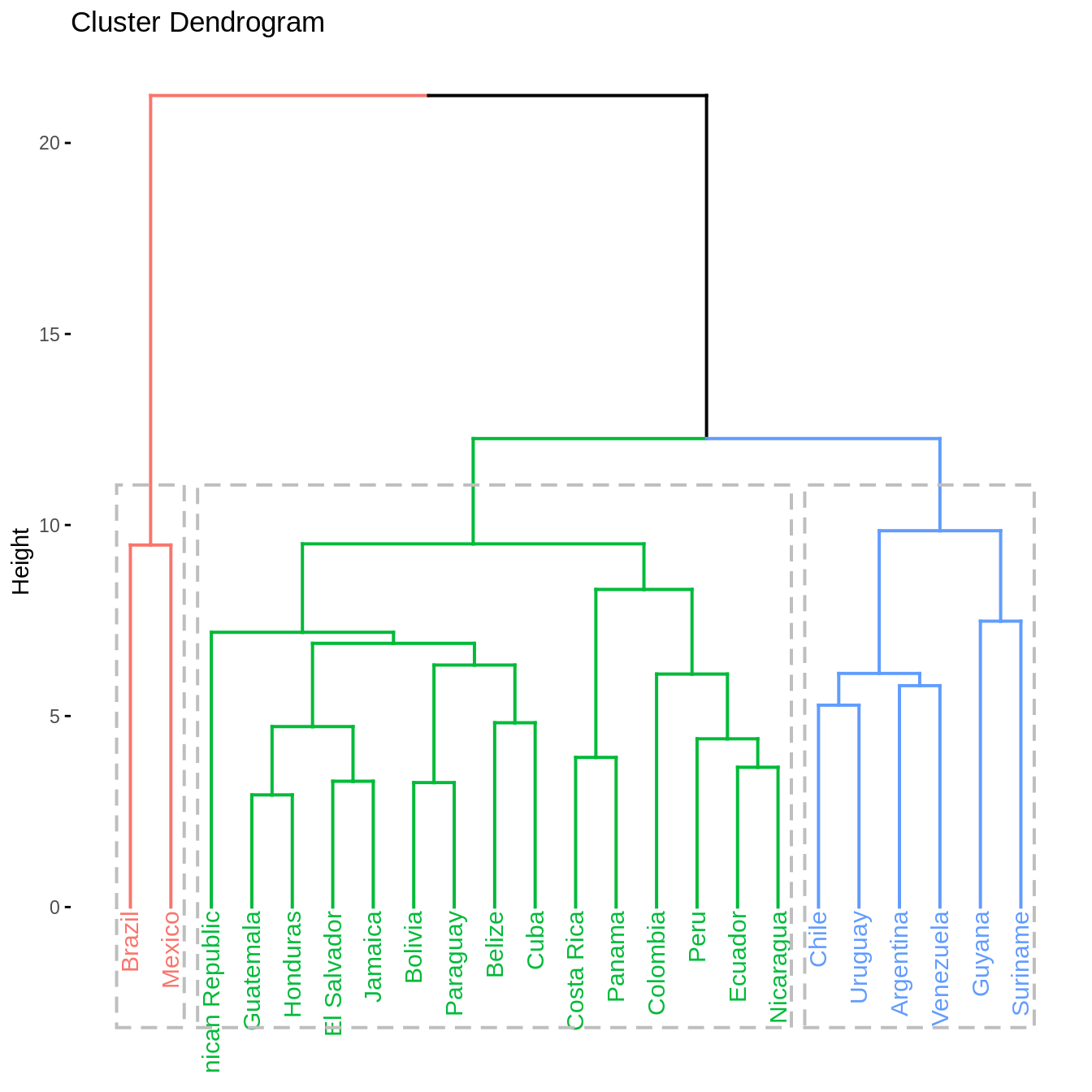# Purpose

In this vignette, we perform some quality control on the database of country socio-hydrologic descriptors. After filtering, the data are examined with a correlation analysis and a clustering analysis.

library("wateReview")
library("ggplot2")
library("factoextra")
#> Welcome! Want to learn more? See two factoextra-related books at https://goo.gl/ve3WBa
library("clValid")
#> Loading required package: cluster

countries_shapefile <- join_database_shapefile(read_countries_database(), read_countries_shapefile())
att_names <- names(countries_shapefile)[-1]

# Visualizing attributes

l.att_map <- lapply(names(countries_shapefile)[-1], make_map)
sample(l.att_map, 1)
#> []
#> Linking to GEOS 3.7.1, GDAL 2.2.2, PROJ 4.9.2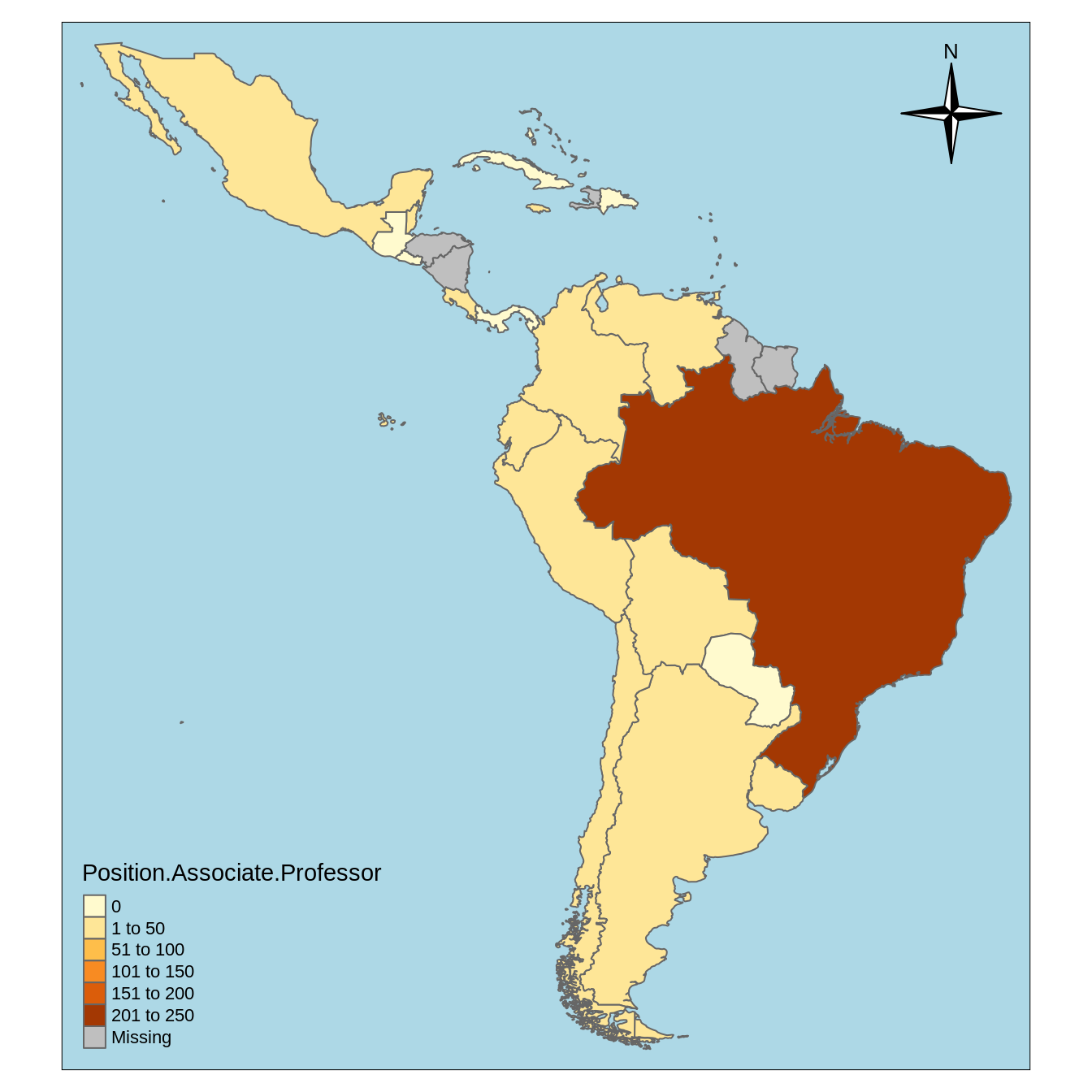l.att_hist <- lapply(names(countries_shapefile)[-1], make_hist)
sample(l.att_hist, 1)
#> []
#> Warning: Removed 13 rows containing non-finite values (stat_density).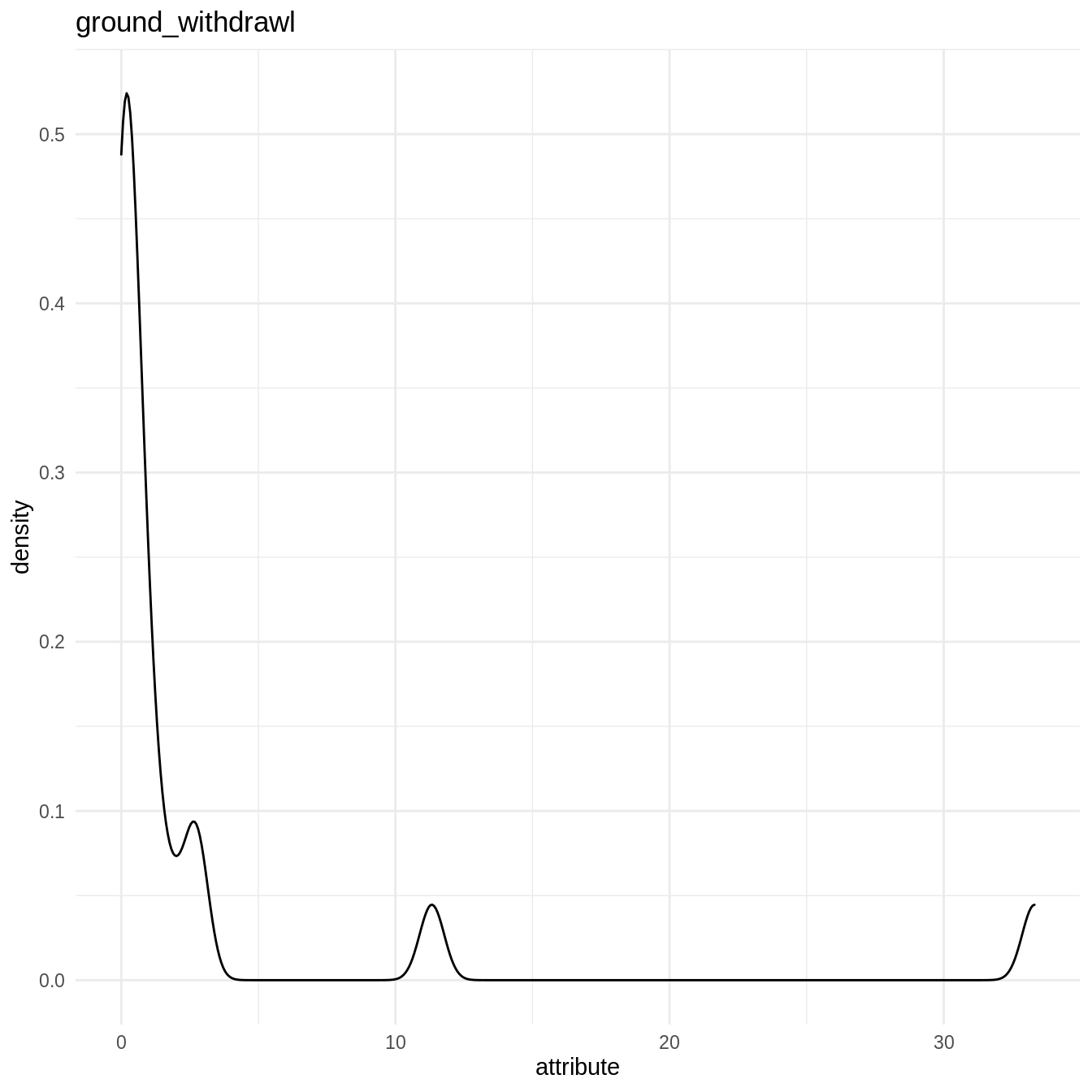# QA/QC on country database

Let’s rescale the data using the make_corrmatrix function with rescale = TRUE. The missmap() function the Amelia package then displays the missing values in the resulting rescaled data.frame.

rescaled_data <- make_corrmatrix(corr_type = "spearman", rescale = TRUE, .plot = FALSE)
rownames(rescaled_data) <- countries_shapefile$COUNTRY Amelia::missmap(rescaled_data)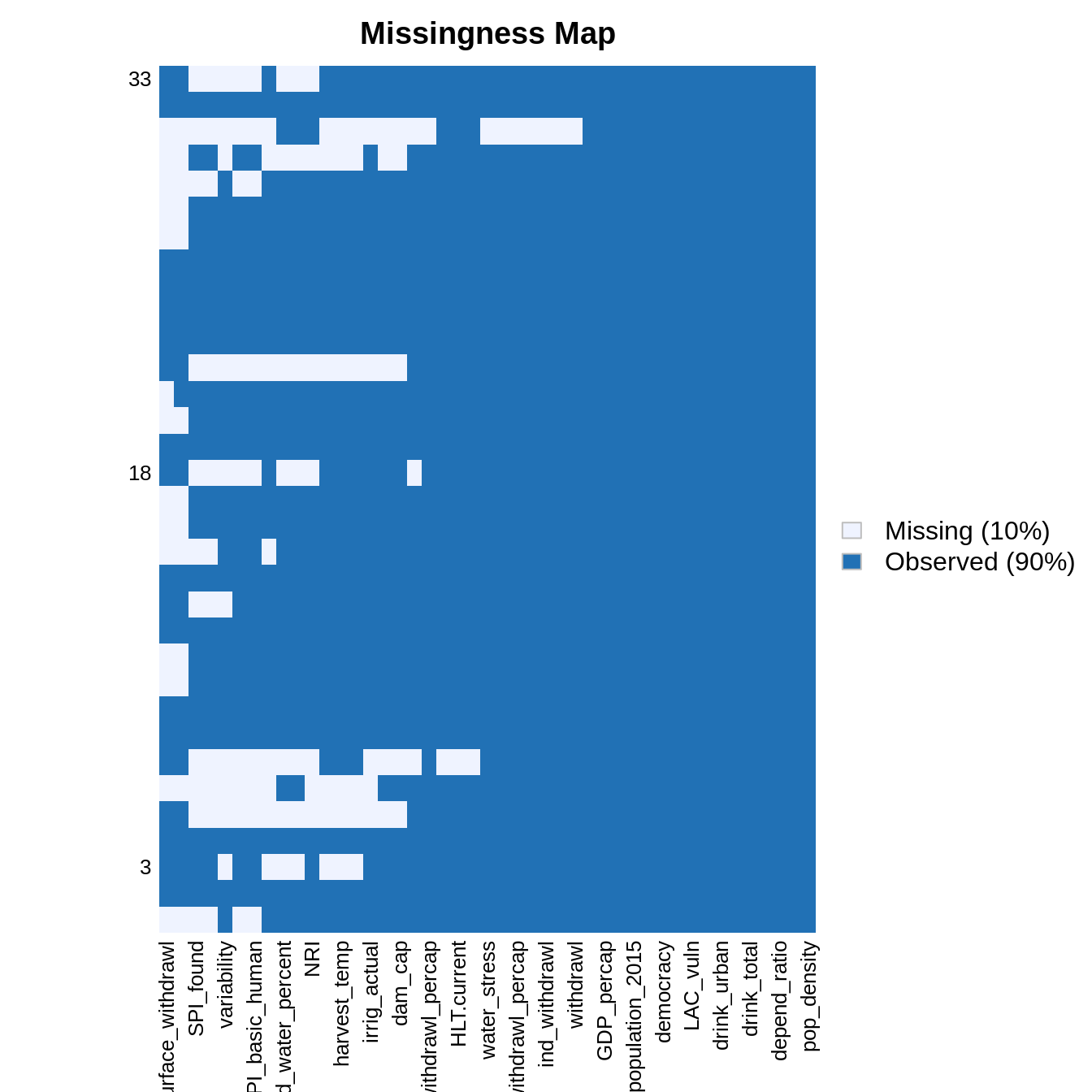Some predictors are missing only in some countries. We now try to identify the best set of predictors and countries by counting the number of NA with count_nas() and varying the threshold of number of NA per countries. count_nas_col <- apply(rescaled_data, MARGIN = 2, FUN = count_nas) count_nas_row <- apply(rescaled_data, MARGIN = 1, FUN = count_nas) names(count_nas_row) <- countries_shapefile$COUNTRY
na_thresholds <- sort(unique(count_nas_row), decreasing = TRUE)
list_count_nas_col <- lapply(na_thresholds, function(na_threshold){
filtered_data <- rescaled_data[count_nas_row <= na_threshold, ]
current_count_nas_col <- apply(filtered_data, MARGIN = 2, FUN = count_nas) # columns
return(current_count_nas_col)
})
count_nas_col_df <- do.call(cbind, list_count_nas_col)
list_country_left <- sapply(na_thresholds, function(na_threshold){
filtered_data <- rescaled_data[count_nas_row <= na_threshold, ]
return(nrow(filtered_data))
})
par(mfrow = c(2, 1))
oce::imagep(t(count_nas_col_df), col = oce::oce.colorsViridis(), decimate = FALSE, xlab = "NA threshold", ylab = "Country descriptors", main = "", cex = 1)
plot(list_country_left, xlab = "NA threshold", ylab = "Number of countries left")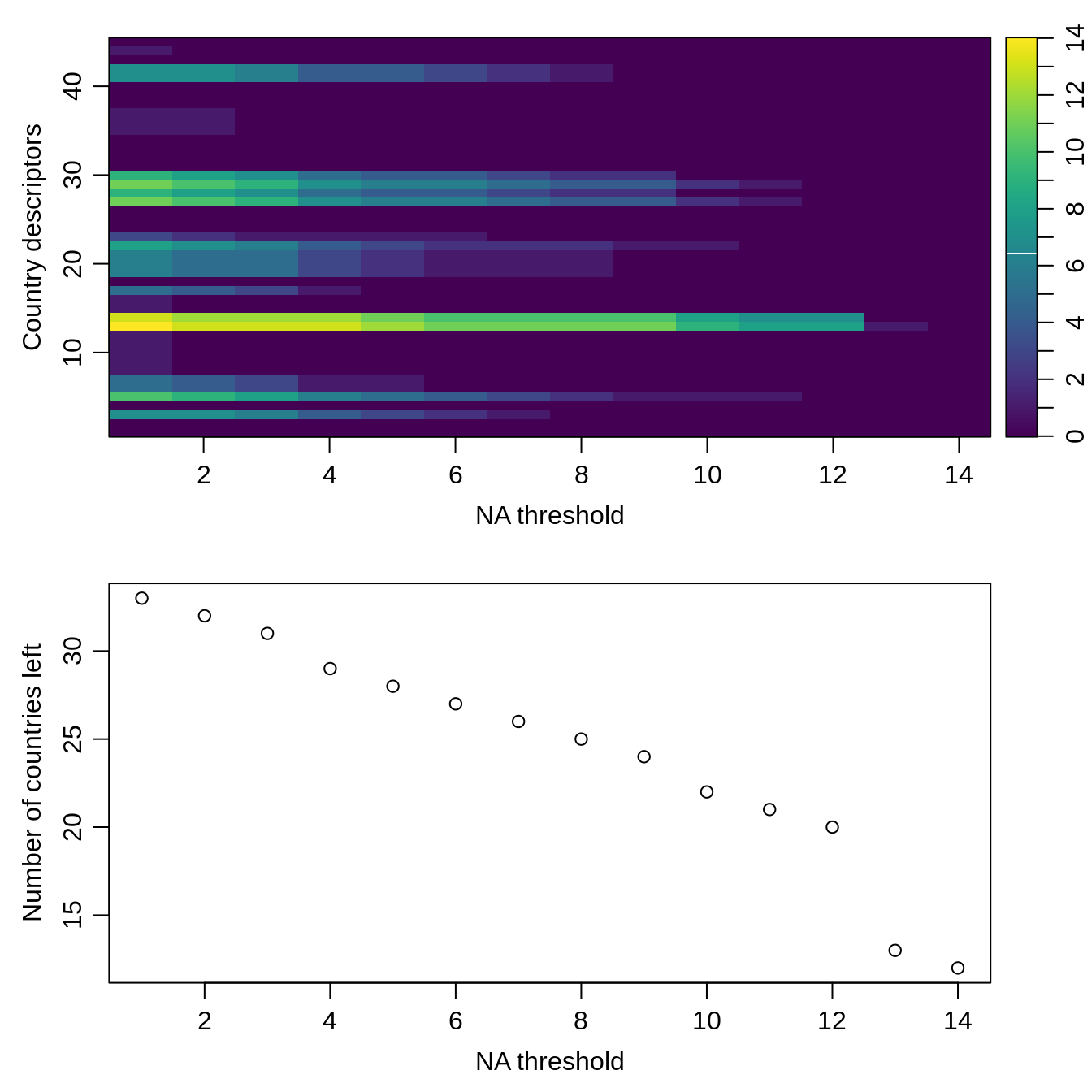Based on this visualization, we filter the country descriptors and drop the countries with missing values. Notice that these removed countries correspond to Carribean nations.

drops <- c("variability", "surface_withdrawl", "ground_withdrawl", "SPI..2017.", "SPI_basic_human", "SPI_found", "SPI_opp")
rescaled_data <- rescaled_data[, !colnames(rescaled_data) %in% drops]
count_nas_row <- apply(rescaled_data, MARGIN = 1, FUN = count_nas) # lines
rownames(rescaled_data) <- countries_shapefile$COUNTRY # countries left rownames(rescaled_data[count_nas_row <= 0, ]) #>  "Argentina" "Belize" "Bolivia" #>  "Brazil" "Chile" "Colombia" #>  "Costa Rica" "Cuba" "Dominican Republic" #>  "Ecuador" "El Salvador" "Guatemala" #>  "Guyana" "Honduras" "Jamaica" #>  "Mexico" "Nicaragua" "Panama" #>  "Paraguay" "Peru" "Suriname" #>  "Uruguay" "Venezuela" # countries removed setdiff(countries_shapefile$COUNTRY, rownames(rescaled_data[count_nas_row <= 0, ]))
#>   "Antigua and Barbuda"            "Bahamas"
#>   "St. Kitts and Nevis"            "St. Lucia"
filtered_data <- rescaled_data[count_nas_row <= 0, ]
Amelia::missmap(filtered_data)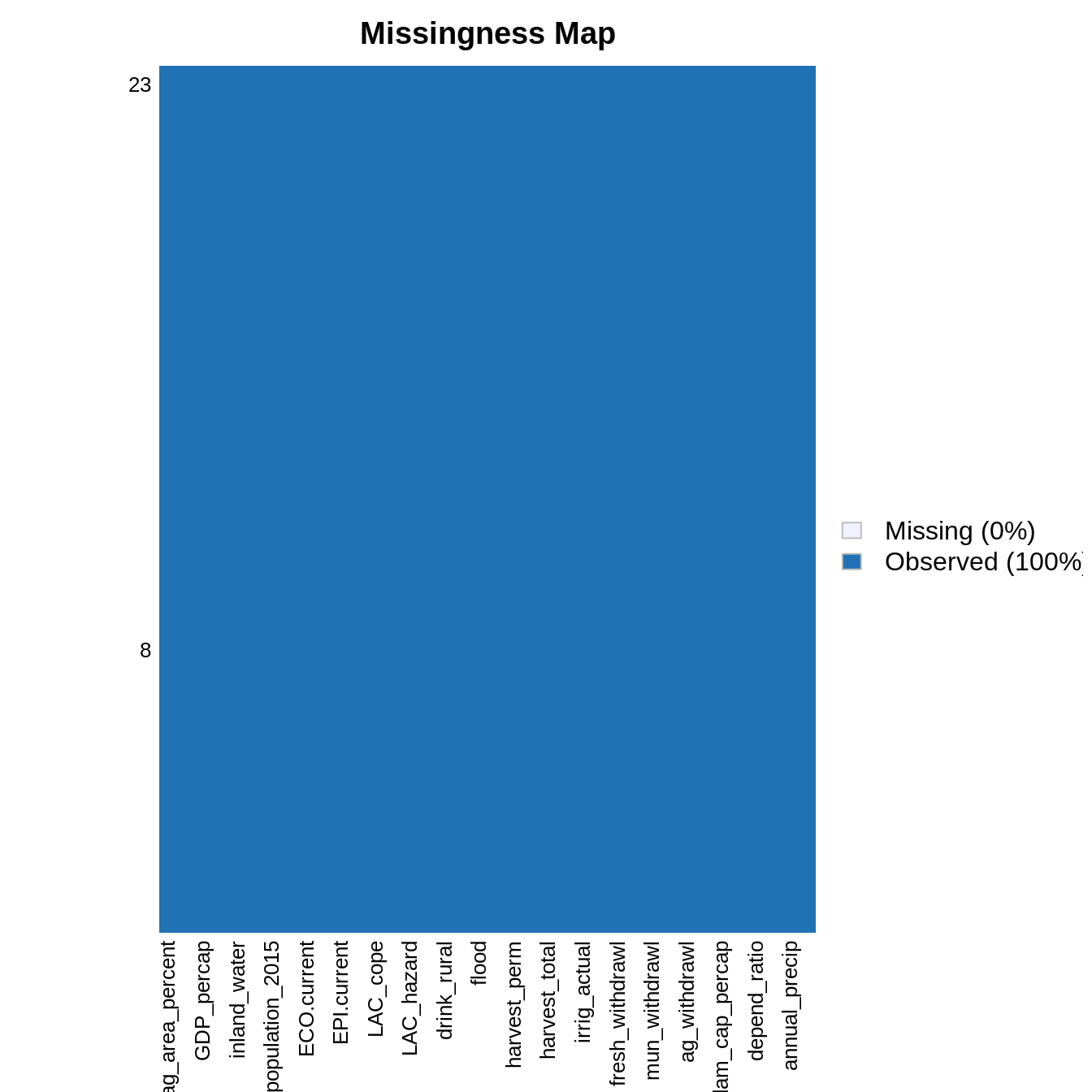# Correlation analysis

We can now calculate the correlations and distance matrices for all types of data.

## Pearson’s correlations

mat <- as.matrix(filtered_data)
correlations <- Hmisc::rcorr(mat, type = "pearson")
correlations$r[which(correlations$P > 0.05)] <- 0
corrplot::corrplot(correlations$r, type = "upper")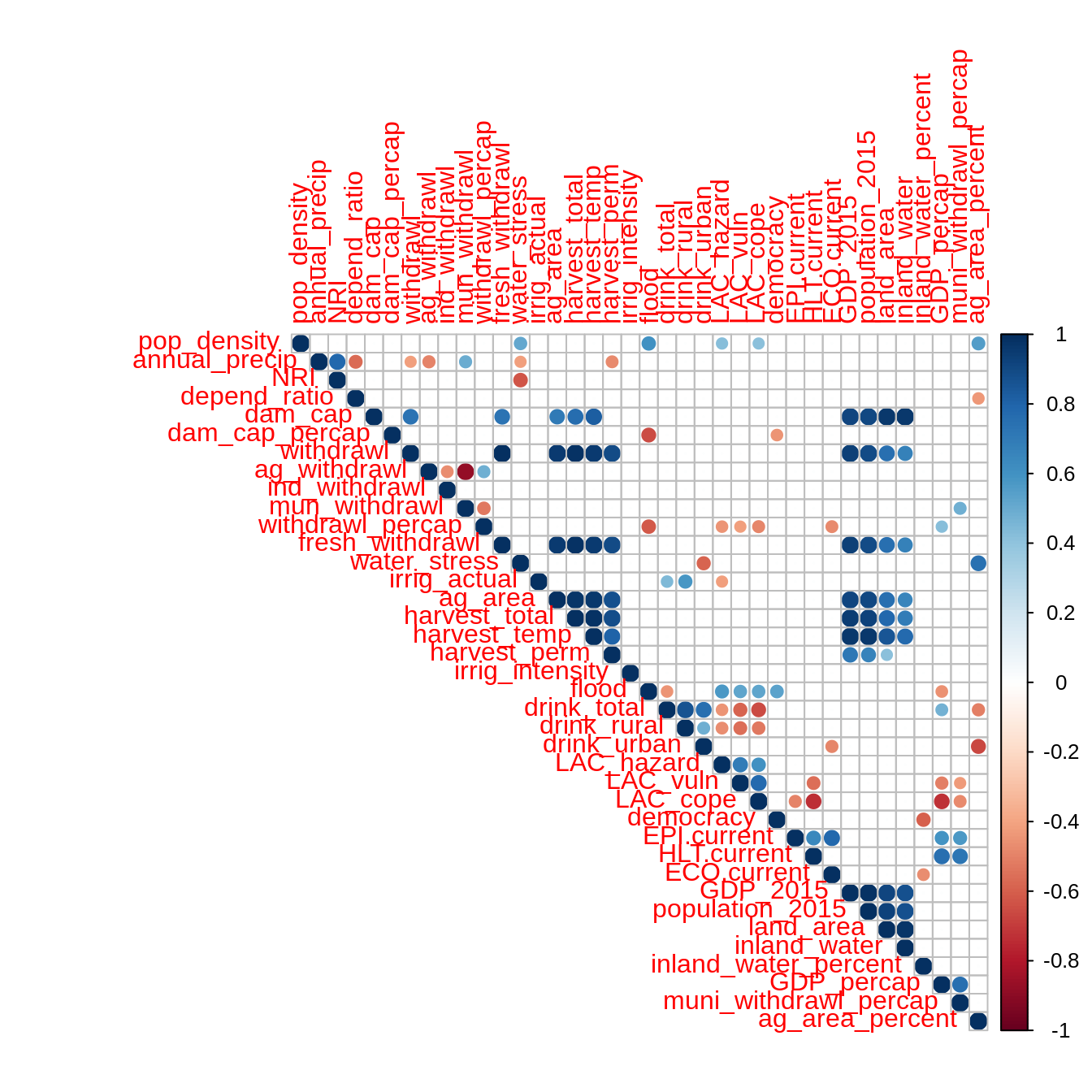## Spearman’s correlations mat <- as.matrix(filtered_data) correlations <- Hmisc::rcorr(mat, type = "spearman") correlations$r[which(correlations$P > 0.05)] <- 0 corrplot::corrplot(correlations$r, type = "upper")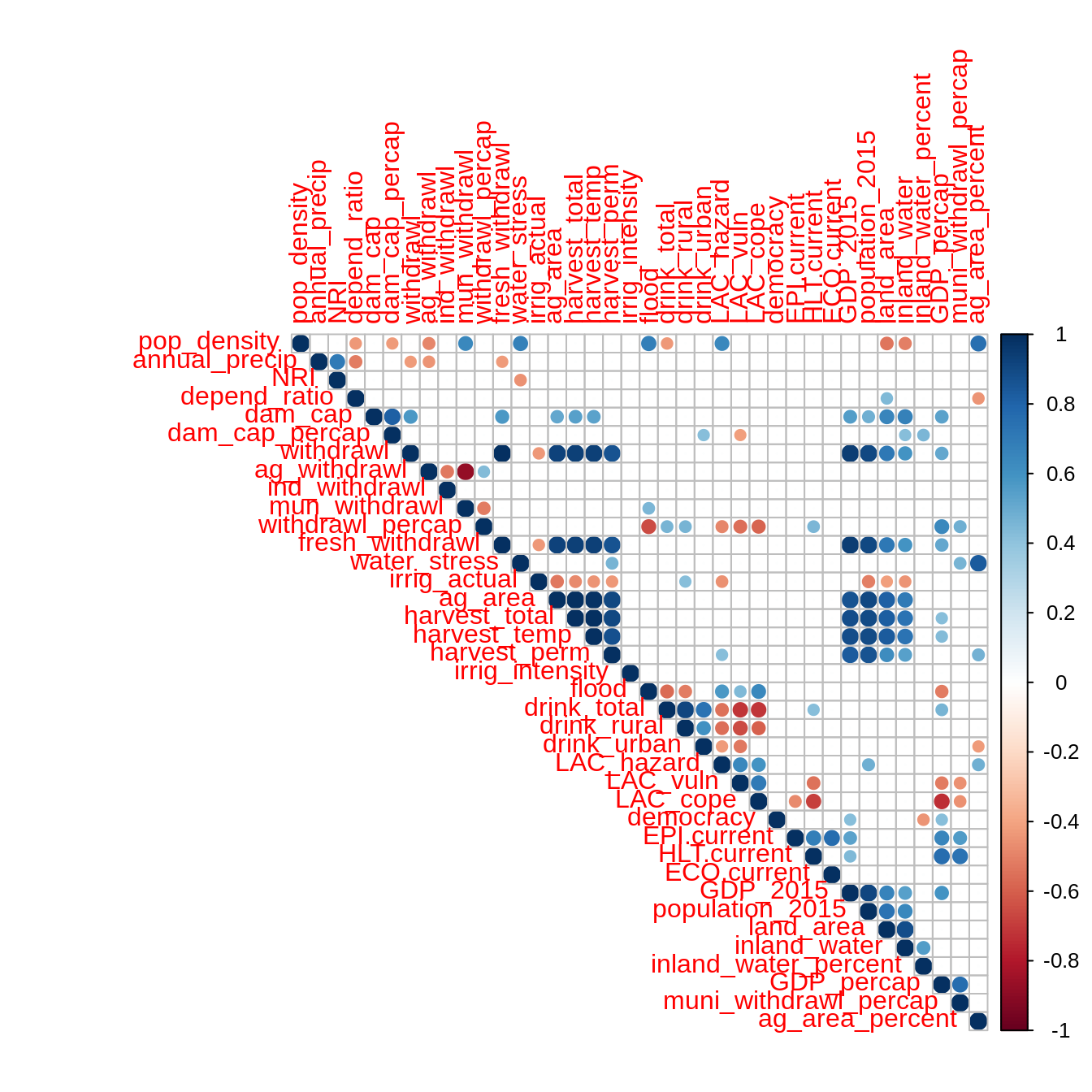## Distance matrices

distance <- get_dist(filtered_data )
p_dist <- fviz_dist(distance, gradient = list(low = "steelblue",  high = "white"), order = TRUE)
p_dist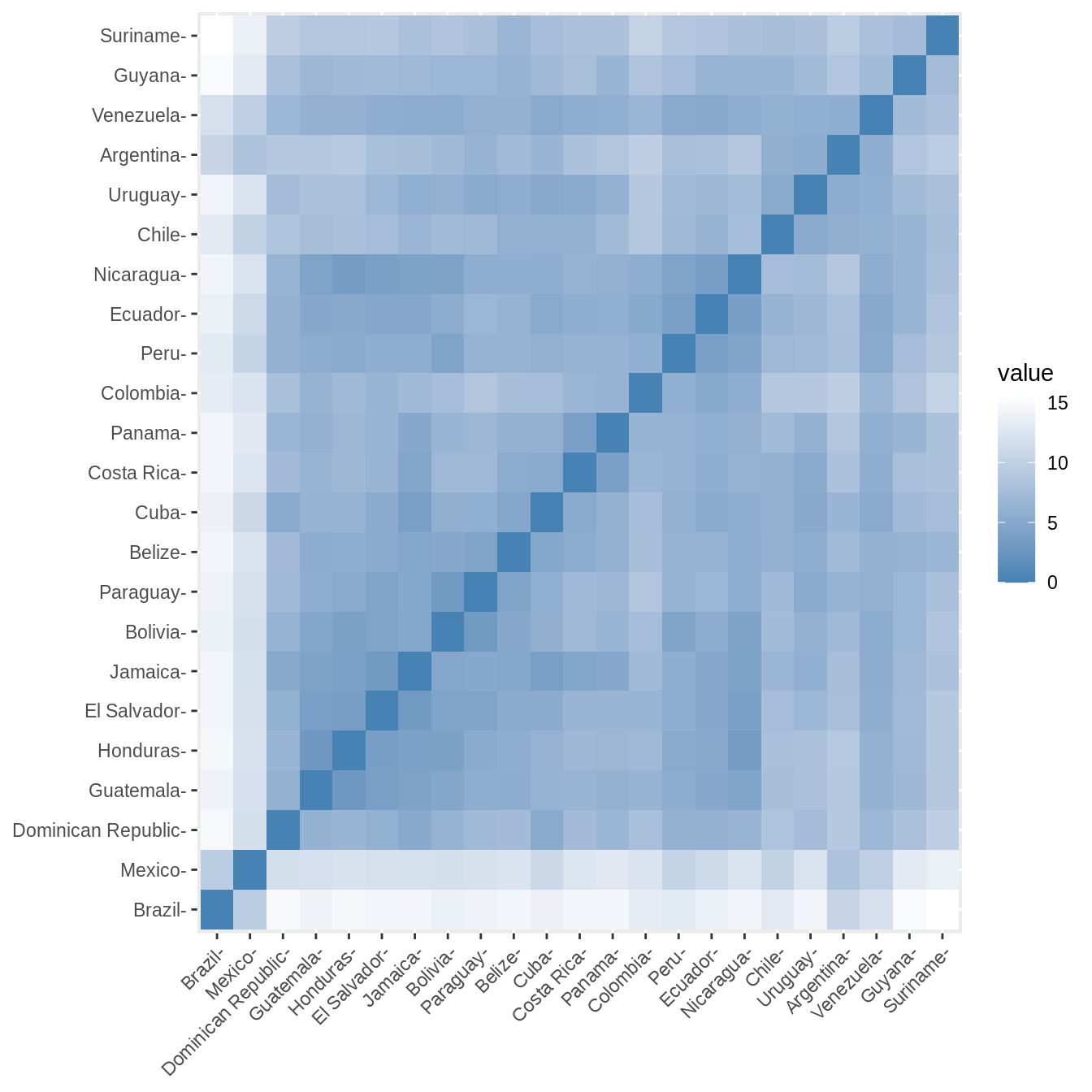# Clustering analysis

Countries in the study area are clustered in relation with socio-hydrologic variables. Two methods of clustering are used: $$k$$-means clustering (Hartigan and Wong 1979) and hierarchical clustering (Murtagh 1983). The clustering is performed with Euclidian distances and following Ward’s criterion. The optimal number of clusters is investigated by evaluating the evolution with the number of clusters of the total within sums of square and of the average silhouette width (Rousseeuw 1987). In addition, the following four validation metrics are used to assess the stability of the clustering under the complete set of clustering variables and a iterative procedure where one variable is removed from the set, an approach akin to leave-one-out cross-validation:

1. the average proportion of (APN) measures the proportion of observations not placed in the same cluster under both cases and evalutes how robust are the clusters under cross-validation (Datta and Datta 2003);
2. the average distance between means (ADM) measures the variation of the cluster center and evaluates the stability of the localization of the cluster in the multi-dimensional clustering variable space (Datta and Datta 2003);
3. average distance (AD) measures the distance between observations placed in the same cluster and evaluates within-cluster stability (Datta and Datta 2003);
4. the figure of merit (FOM) estimates the predictive power of the clustering algorithm by measuring the within-cluster variance of the removed variable (Yeung, Haynor, and Ruzzo 2001).

Both clustering methods yield similar results. The total within sum of squares exhibits a shift in the evolution of the total within sum of square after two clusters are chosen. Similarly, the average silhouette width strongly exhibits a peak for two clusters. Further inspection of clustering in PCA dimensions indicates that the cluster with Mexico and Brazil is significantly distinct from all other countries, explaining the observation of a sharp peak in average silhouette width. However, validation metrics exhibits optimal null values of APN and ADM for two or three clusters. In addition, AD and FOM are lower for three clusters than for two. Based on this results, we chose three clusters to describe the grouping of countries based on their socio-hydrologic variables.

## Optimum clustering

We now derive the optimum clustering for $$k$$-means and hierarchical clustering.

### $$k$$-means

get_optimk(filtered_data, method = "kmeans")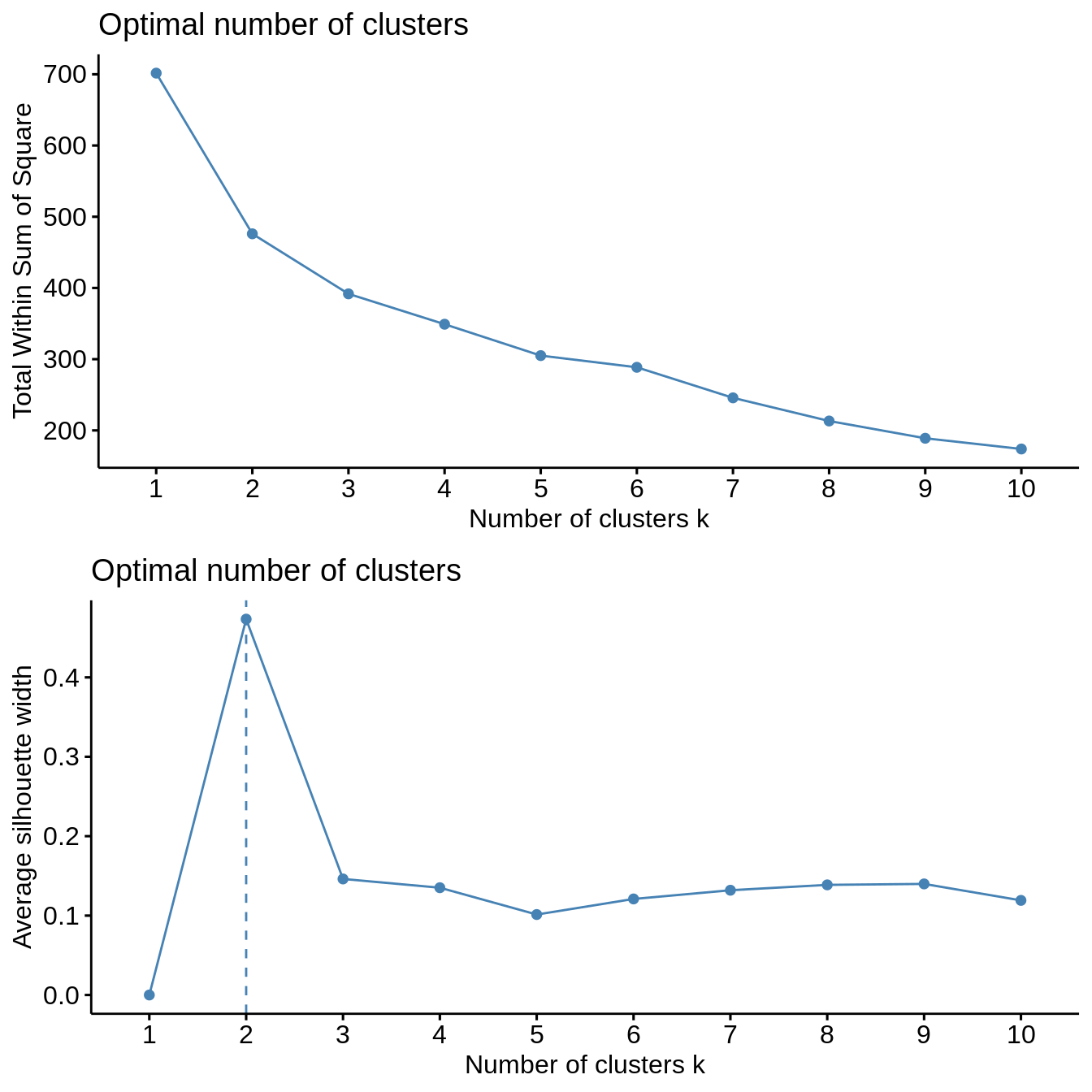### Hierarchical clustering

get_optimk(filtered_data, method = "hierarchical")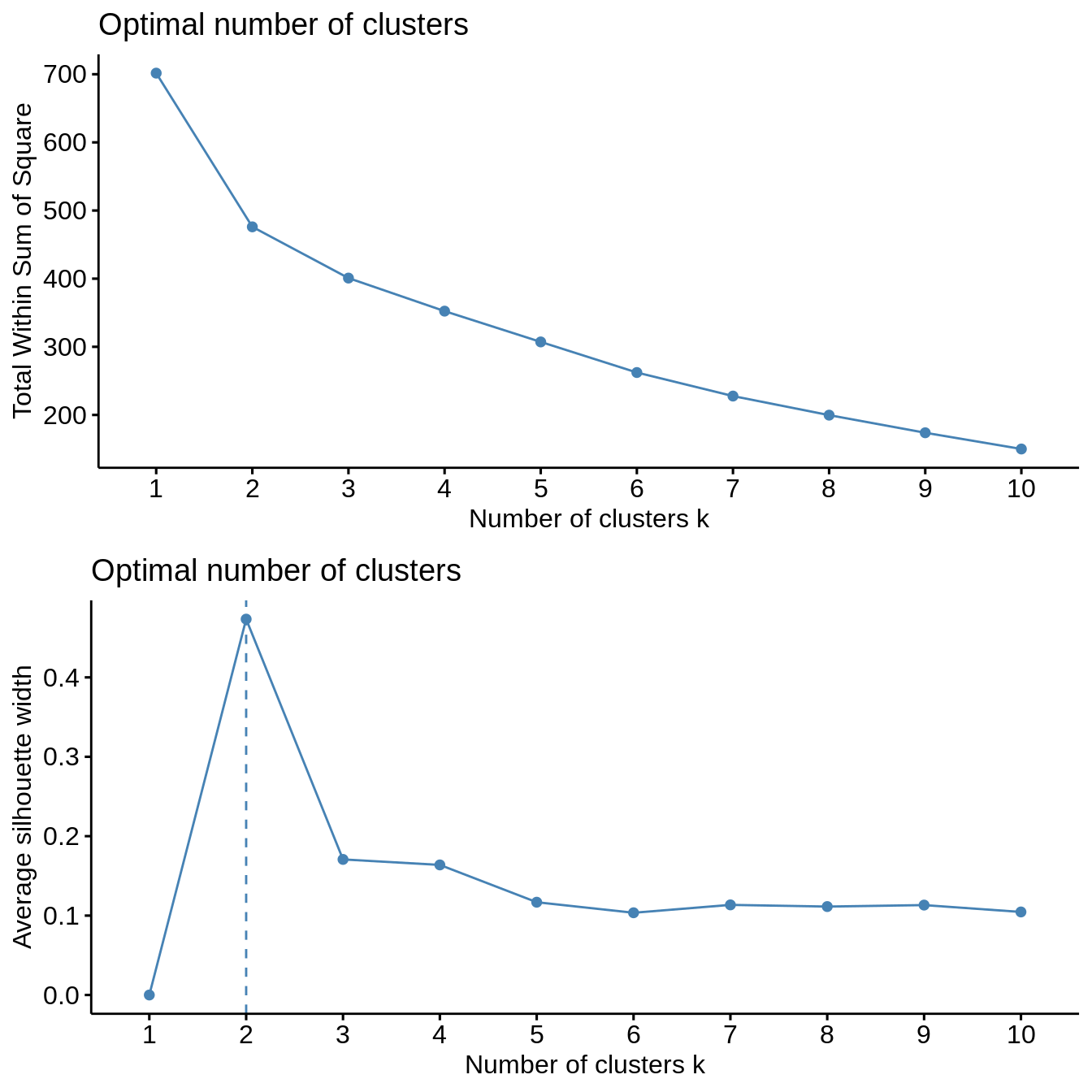## Clustering stability

### $$k$$-means

cl_stability <- clStab(filtered_data, method = "kmeans")
stab <- cl_stability$stab optim_df <- cl_stability$optim_df
summary(stab)
#>
#> Clustering Methods:
#>  kmeans
#>
#> Cluster sizes:
#>  2 3 4 5 6 7 8 9 10
#>
#> Validation Measures:
#>                  2      3      4      5      6      7      8      9     10
#>
#> kmeans APN  0.0022 0.0000 0.0562 0.0702 0.1154 0.1833 0.1219 0.0912 0.1072
#>        AD   5.9933 5.5334 4.9773 4.5944 4.3233 4.1371 3.5719 3.1712 2.9348
#>        ADM  0.0237 0.0000 0.4368 0.3939 0.5818 1.3910 0.7739 0.5986 0.6711
#>        FOM  0.7431 0.7033 0.6818 0.6633 0.6682 0.6501 0.6389 0.6244 0.6135
#>
#> Optimal Scores:
#>
#>     Score  Method Clusters
#> APN 0.0000 kmeans 3
#> FOM 0.6135 kmeans 10
print("Optimal Number of Cluster under One Std Rule")
#>  "Optimal Number of Cluster under One Std Rule"
print(optim_df)
#>   measure cluster
#> 1     APN       2
#> 4     FOM       7

### Hierarchical clustering

cl_stability <- clStab(filtered_data, method = "hierarchical")
stab <- cl_stability$stab optim_df <- cl_stability$optim_df
summary(stab)
#>
#> Clustering Methods:
#>  hierarchical
#>
#> Cluster sizes:
#>  2 3 4 5 6 7 8 9 10
#>
#> Validation Measures:
#>                        2      3      4      5      6      7      8      9     10
#>
#> hierarchical APN  0.0000 0.0000 0.0041 0.0400 0.0505 0.0512 0.0677 0.0815 0.0828
#>              AD   5.9857 5.5736 5.1332 4.7328 4.3883 3.9961 3.6887 3.2904 3.0251
#>              ADM  0.0000 0.0000 0.0316 0.3577 0.4152 0.3476 0.4170 0.6353 0.5779
#>              FOM  0.7376 0.7056 0.7033 0.6737 0.6643 0.6493 0.6465 0.6264 0.6129
#>
#> Optimal Scores:
#>
#>     Score  Method       Clusters
#> APN 0.0000 hierarchical 2
#> FOM 0.6129 hierarchical 10
print("Optimal Number of Cluster under One Std Rule")
#>  "Optimal Number of Cluster under One Std Rule"
print(optim_df)
#>   measure cluster
#> 1     APN       2
#> 4     FOM       7

## Cluster visualization

### $$k$$-means

k2 <- kmeans(filtered_data, centers = 3, nstart = 25)
fviz_cluster(k2, geom = "text",  data = filtered_data) + cowplot::theme_cowplot()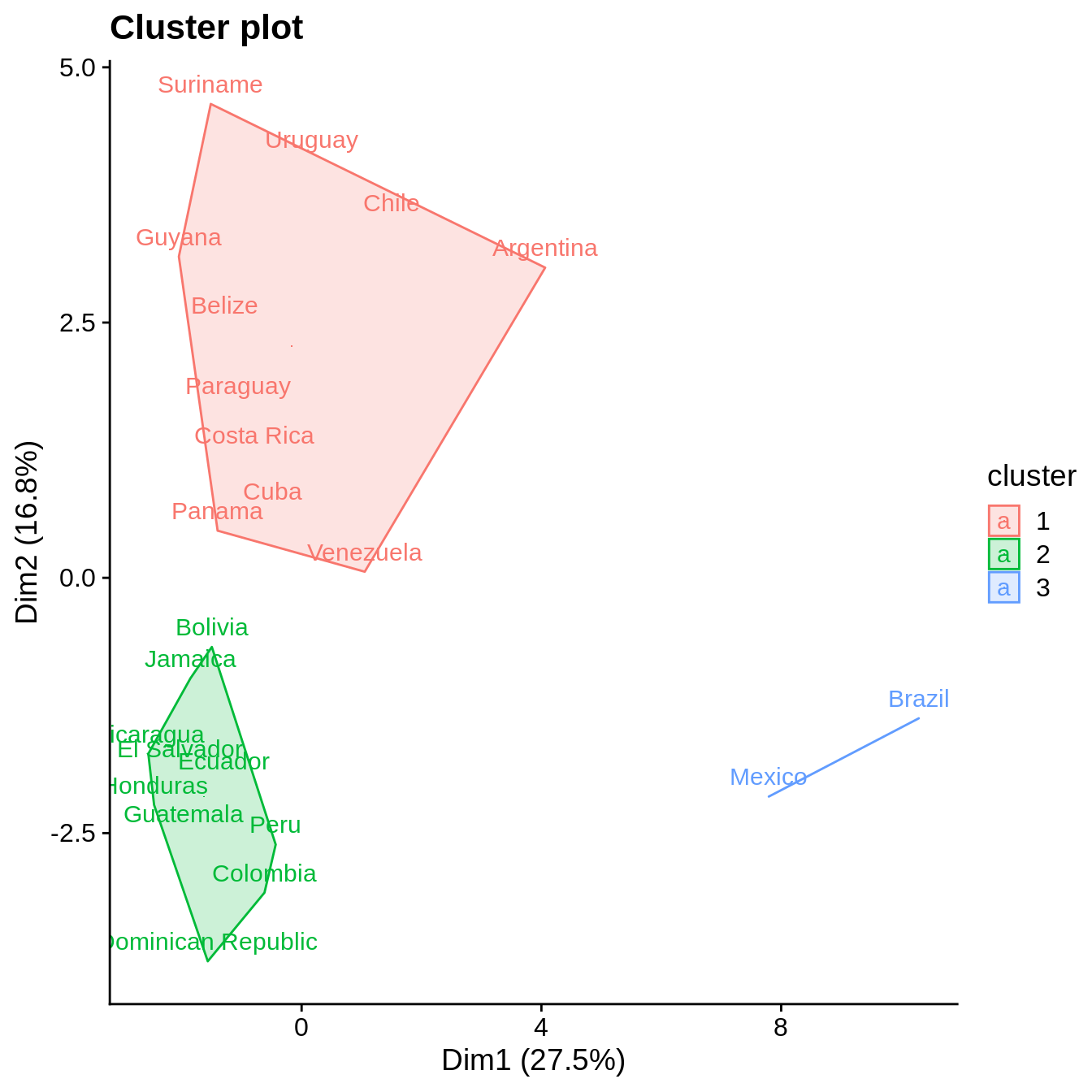### Hierarchical clustering

res.hc <- eclust(filtered_data, "hclust", k = 3, graph = FALSE)
fviz_dend(res.hc, rect = TRUE, show_labels = TRUE)